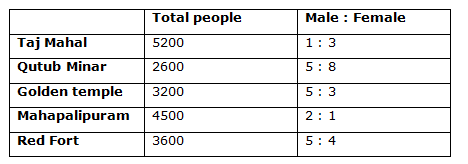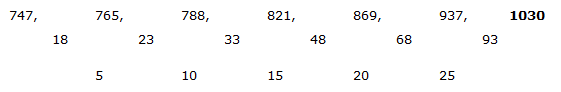# “20-20” Quantitative Aptitude | Crack Indian bank PO Prelims 2018 Day-213

Dear Readers, Find the aptitude test questions to crack latest bank exams. We regularly provide 20 aptitude test questions daily for students. Aspirants practice these questions on a regular basis to improve your score in aptitude section. Aspirants preparing for the exams can make use of this “20-20” Quantitative Aptitude Questions. Here we have started New Series of Practice Materials specially for Crack Indian bank PO  Prelims 2018. Aspirants those who are preparing for the exams can use this “20-20” Quantitative Aptitude Questions.

[WpProQuiz 3862]

Click “Start Quiz” to attend these Questions and view Solutions

Directions (Q. 1 – 5): What value should come in the place of question mark (?) in the following number series?

1) 11, 32, 126, 627, 3758, ?

1. 10563
2. 15784
3. 18247
4. 26301
5. 20489

2) 71, 248.5, 869.75, ? , 10654.4375

1. 3044.125
2. 1278.25
3. 1896.325
4. 2784.5
5. 22356.75

3) 34, 42, 111, 397,? , 8781

1. 785
2. 942
3. 826
4. 1527
5. 1713

4) 1127, 1248, 2976, 3145, 5889, ?

1. 6028
2. 6114
3. 6576
4. 6320
5. 6472

5) 747, 765, 788, 821, 869, 937, ?

1. 984
2. 1016
3. 1030
4. 1178
5. 1452

Directions (Q. 6 – 10): What value should come in the place of question mark (?) in the following questions?

6) 12 ¾ % of 2400 + 23 ½ % of 800 + 452 = 1125 ÷ 25 + 4 *?

1. 658.5
2. 532.5
3. 776.5
4. 618.5
5. 590.5

7) (12/75) of (48/1136) of (32/96) of 10650 =? % of 500

1. 8
2. 2
3. 4
4. 6
5. None of these

8) 15 1/3 – 4 1/6 + 8 5/12 – 7 2/3 =? + 2 7/12

1. 11 2/5
2. 13 ¼
3. 8 3/7
4. 9 1/3
5. None of these

9) (6390 ÷ 15) + (7182 ÷ 19) + (10224 ÷ 8) =? – 24 % of 750

1. 2534
2. 2262
3. 2876
4. 3148
5. None of these

10) 157464 + √8836 – 45 % of 800 =? + (1188 ÷ 9) – 362

1. 952
2. 816
3. 878
4. 924
5. None of these

11) A and B invested in the ratio of 15: 14. The profits are divided in the ratio of 3: 4. If A has invested for 7 months, then B invested for?

1. 7 months
2. 6 months
3. 10 months
4. 9 months
5. None of these

12) The simple interest on a sum of money is 1/8 of the sum. If the number of years is equal to twice the rate percent per annum, then the rate percent per annum is?

1. 2 ½ %
2. 4 %
3. 5 %
4. 3 1/4 %
5. None of these

13) Out of 12 students studying in a class, 4 from Madhya Pradesh, 5 from Tamil Nadu and 3 from Delhi. Three students are to be selected at random. What are the chances that at least one is from Delhi?

1. 32/57
2. 28/63
3. 11/34
4. 34/55
5. None of these

14) A Loss of Rs. 1500 is made by selling an article if one-fourth of it is sold at 12% profit and the remaining at 8 % loss. What is the total cost price of the article?

1. 39000
2. 50000
3. 44000
4. 46000
5. None of these

15) A boat can travel 9.6 km downstream in 32 minutes. If the speed of the current is 1/5 of the speed of the boat in still water, then find the speed of upstream?

1. 8 km/hr
2. 10 km/hr
3. 12 km/hr
4. 16 km/hr
5. None of these

Directions (Q. 16 – 20) Study the following information carefully and answer the given questions:

Following table shows the total number of people went for a different famous place in a certain week and the ratio of male and female also given.16) Total number of people visited Taj Mahal and Golden Temple together is approximately what percentage of total number of people visited Qutub Minar and Red Fort together?

1. 120 %
2. 135 %
3. 127 %
4. 102 %
5. 94 %

17) Find the ratio between the total number of male visited Mahapalipuram to that of total number of female visited Golden temple?

1. 5 : 2
2. 3 : 1
3. 7 : 5
4. 9 : 7
5. None of these

18) Find the difference between the total number of male visited Qutub Minar and Red Fort together to that of female visited the same places?

1. 270
2. 300
3. 200
4. 350
5. 420

19) Find the average number of female visited Taj Mahal and Red Fort together?

1. 2180
2. 2230
3. 2870
4. 2750
5. None of these

20) Total number of people visited Mahapalipuram is what percentage of total number of people visited Red Fort?

1. 114 %
2. 125 %
3. 136 %
4. 148 %
5. 103 %

Direction (1-5) :

The pattern is, *3 – 1, *4 – 2, *5 – 3, *6 – 4, *7 – 5,..

The pattern is, *3.5

The pattern is, *1 + 23, *2 +33, *3 + 43, *4 + 53, *5 + 63,..

The pattern is, +112, +123, +132, +143, +152, +163,..The difference of difference is, 5, 10, 15, 20, 25,..

Direction (6-10) :

(51/400)*2400 + (47/200)*800 + 2025 = (1125/25) + 4x

306 + 188 + 2025 – 45 = 4x

2474 = 4x

X = 2474/4 = 618.5

(12/75)* (48/1136)*(32/96) * 10650 = (x/100)*500

24 = 5x

X = 24/5 = 4.8

15 1/3 – 4 1/6 + 8 5/12 – 7 2/3 – 2 7/12 = x

(15 – 4 + 8 – 7 – 2) (1/3 – 1/6 + 5/12 – 2/3 – 7/12) = x

10 [(4 – 2 + 5 – 8 – 7)/12] = x

X = 10 (-8/12) = 10 (-2/3)

X = (30 – 2)/3 = 28/3 = 9 1/3

(6390 ÷ 15) + (7182 ÷ 19) + (10224 ÷ 8) = x – (24/100)*750

426 + 378 + 1278 = x – 180

426 + 378 + 1278 + 180 = x

X = 2262

54 + 94 – (45/100)*800 = x + (1188/9) – 1296

54 + 94 – 360 + 1296 = x + 132

54 + 94 – 360 + 1296 – 132 = x

X = 952

Investment ratio = 15:14

Profits = 3:4, A has invested for 7 months.

Ratio of Profit = 15*7: 14*x = 15: 2x

According to the question,

15/2x = ¾

60 = 6x

X = 10 months

S.I = (1/8) P

(1/8) P = (P × 2r × r) / 100 (Here n=2r)

1/8 = 2r2 / 100

r2 = 50/8 = 25/4

r = 5/2 = 2 ½ %

Total probability = 12C3

Required probability= 1- none is from Delhi

None is from Delhi,

= > 9C3

Required probability= 1- none is from Delhi

= > 1 – (9C3 / 12C3)

= > 1 – (21/55)

= > 34/55

Let cost price be x,

Loss = 1500 = Cp – Sp

X – [X*(1/4)*(112/100) + x*(3/4)*(92/100)] = 1500

X – (28x + 69x)/100= 1500

100x – 97x = 150000

3x = 150000

X = Rs. 50000

Let the speed of the boat in still water be x km/hr

Speed of the current = x/5

Speed of downstream = x + x/5= 6x/5

Speed of downstream = 9.6/(32/60) = 9.6*(60/32)= 18 km/hr

So,

6x/5= 18

x = 15 km/hr

Speed of boat in still water = 15 km/hr

Speed of current = 15/5 = 3 km/hr

Speed of upstream = 15 – 3 = 12 km/hr

Direction (16-20) :

Total number of people visited Taj Mahal and Golden Temple together

= > 5200 + 3200 = 8400

Total number of people visited Qutub Minar and Red Fort together

= > 2600 + 3600 = 6200

Required % = (8400/6200)*100 = 135 %

The total number of male visited Mahapalipuram

= > 4500*(2/3) = 3000

The total number of female visited Golden temple

= > 3200*(3/8) = 1200

Required ratio = 3000: 1200 = 5: 2

The total number of male visited Qutub Minar and Red Fort together

= > 2600*(5/13) + 3600*(5/9)

= > 1000 + 2000 = 3000

The total number of female visited Qutub Minar and Red Fort together

= > 2600*(8/13) + 3600*(4/9)

= > 1600 + 1600 = 3200

Required difference = 3200 – 3000 = 200

The total number of female visited Taj Mahal and Red Fort together

= > 5200*(3/4) + 3600*(4/9)

= > 3900 + 1600 = 5500

Required average = 5500/2 = 2750